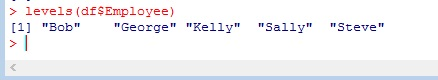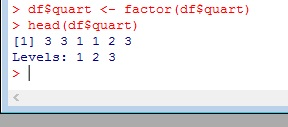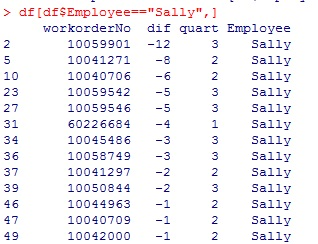# R: Filter Dataframes

When working with large data sets, often you only want to see a portion of the data. If you are running a query on the graduating class of a high school, you don’t want your results to be clogged up with hundreds of freshmen, sophomores, juniors. To accomplish this, we use filtering. Filtering a dataframe is a very useful skill to have in R. Luckily R makes this task relatively simple.

Import the data

```df <- read.csv(file.choose())

Let’s take a look at it. We have a data set with 4 columns, workorderNo – Work Order Number, dif – change code since last work order reading, quart – quarter (referring to calendar year), Employee – employee name.The first thing we need to know when dealing with dataframes is how to use the \$

In R, the “\$” placed between a dataframe name and a column name signify which column you want to work with.

```head(df\$Employee)Notice the line that says Levels: after the Employee list. This lets you know this column is a factor. You can see all the unique elements (factors) in your list by using the levels() command.

`level(df\$Employee)`Taking another look at our data, the quart column consists of 3 numbers (1,2,3) representing quarters of the year. If you think back to your statistics classes, these numbers are ordinal (meaning they only provide a ranking – you will never use them in a calculation – quart 2 + quart 3 has no real meaning.) So we should make this column a factor as well.

R makes that easy to do.

```df\$quart <- factor(df\$quart)Okay, now onto filtering. Let’s see how easy it can be.

First we assign our filtering logic to a variable, then we place our variable inside the dataframe’s square brackets []

```filter <- df\$quart==2
df[filter,]```Or you can just put your logic directly in the dataframe’s square brackets[]

`df[df\$Employee=="Sally",]`

Now we just see SallyWe can use an and “&” operator to filter by Sally and quart 1

`df[df\$Employee=="Sally" & df\$quart == 1,]`